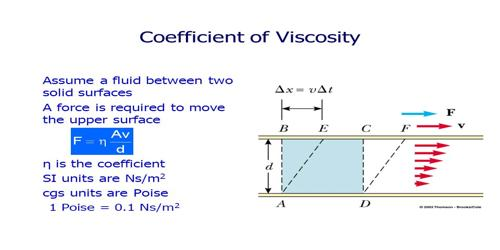Physics

# Co-efficient of ViscosityCo-efficient of Viscosity

Definition: For unit velocity gradient the amount of viscous force that acts per unit area of a fluid is called co-efficient of viscosity of that fluid. This force acts along tangent to the layers of the fluid.

or, The tangential force needed per unit area to maintain unit velocity gradient is a liquid is called co-efficient of viscosity of that liquid.

Dimension and unit of co-efficient of viscosity: We know.

F = η.A. dv/dy

or, η = (F/A) (dv/dy)

Dimension, η = (Dimension of F/Dimension of area) / (Dimension of distance/Dimension of Velocity)

= [(MLT-2 x L) / (L2 x L/T)]

= [(MLT-2 x L x T) / L3] = [ML-1T-1]

Unit of η: In M.K.S. and S.I. method, the unit of η is Nsm-2. In many cases poise is used as the unit of η. 10 poise = 1 Nam-2.

“Co-efficient of viscosity 1 Nsm-2 – This statement means that 1 N tangential force is required to maintain a relative velocity of I ms-1 between two layers of a liquid having 1 m2 area and a distance of 1 m. Coefficient of viscosity is sometimes called dynamic viscosity.